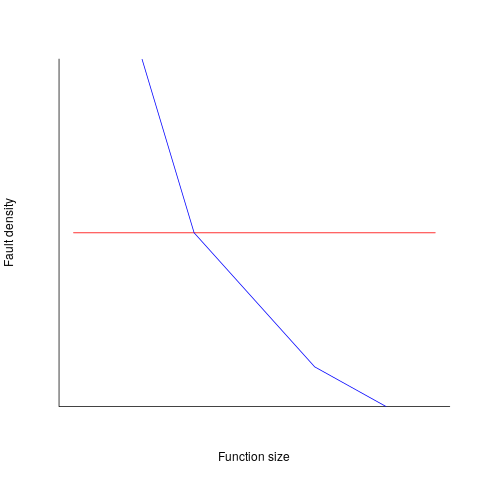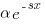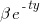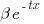## Impact of function size on number of reported faults

Are longer functions more likely to contain more coding mistakes than shorter functions?

Well, yes. Longer functions contain more code, and the more code developers write the more mistakes they are likely to make.

But wait, the evidence shows that most reported faults occur in short functions.

This is true, at least in Java. It is also true that most of a Java program’s code appears in short methods (in C 50% of the code is contained in functions containing 114 or fewer lines, while in Java 50% of code is contained in methods containing 4 or fewer lines). It is to be expected that most reported faults appear in short functions. The plot below shows, left: the percentage of code contained in functions/methods containing a given number of lines, and right: the cumulative percentage of lines contained in functions/methods containing less than a given number of lines (code+data):Does percentage of program source really explain all those reported faults in short methods/functions? Or are shorter functions more likely to contain more coding mistakes per line of code, than longer functions?

Reported faults per line of code is often referred to as: defect density.

If defect density was independent of function length, the plot of reported faults against function length (in lines of code) would be horizontal; red line below. If every function contained the same number of reported faults, the plotted line would have the form of the blue line below.Two things need to occur for a fault to be experienced. A mistake has to appear in the code, and the code has to be executed with the ‘right’ input values.

Code that is never executed will never result in any fault reports.

In a function containing 100 lines of executable source code, say, 30 lines are rarely executed, they will not contribute as much to the final total number of reported faults as the other 70 lines.

How does the average percentage of executed LOC, in a function, vary with its length? I have been rummaging around looking for data to help answer this question, but so far without any luck (the llvm code coverage report is over all tests, rather than per test case). Pointers to such data very welcome.

Statement execution is controlled by if-statements, and around 17% of C source statements are if-statements. For functions containing between 1 and 10 executable statements, the percentage that don’t contain an if-statement is expected to be, respectively: 83, 69, 57, 47, 39, 33, 27, 23, 19, 16. Statements contained in shorter functions are more likely to be executed, providing more opportunities for any mistakes they contain to be triggered, generating a fault experience.

Longer functions contain more dependencies between the statements within the body, than shorter functions (I don’t have any data showing how much more). Dependencies create opportunities for making mistakes (there is data showing dependencies between files and classes is a source of mistakes).

The previous analysis makes a large assumption, that the mistake generating a fault experience is contained in one function. This is true for 70% of reported faults (in AspectJ).

What is the distribution of reported faults against function/method size? I don’t have this data (pointers to such data very welcome).

The plot below shows number of reported faults in C++ classes (not methods) containing a given number of lines (from a paper by Koru, Eman and Mathew; code+data):It’s tempting to think that those three curved lines are each classes containing the same number of methods.

What is the conclusion? There is one good reason why shorter functions should have more reported faults, and another good’ish reason why longer functions should have more reported faults. Perhaps length is not important. We need more data before an answer is possible.

## How should involved if-statement conditionals be structured?

Which of the following two if-statements do you think will be processed by readers in less time, and with fewer errors, when given the value of `x`, and asked to specify the output?

```// First - sequence of subexpressions
if (x > 0 && x < 10 || x > 20 && x < 30)
print("a");
else
print "b");

// Second - nested ifs
if (x > 0 && x < 10)
print("c");
else if (x > 20 && x < 30)
print("d");
else
print("e");
```

Ok, the behavior is not identical, in that the `else if`-arm produces different output than the preceding `if`-arm.

The paper Syntax, Predicates, Idioms — What Really Affects Code Complexity? analyses the results of an experiment that asked this question, including more deeply nested if-statements, the use of negation, and some for-statement questions (this post only considers the number of conditions/depth of nesting components). A total of 1,583 questions were answered by 220 professional developers, with 415 incorrect answers.

Based on the coefficients of regression models fitted to the results, subjects processed the nested form both faster and with fewer incorrect answers (code+data). As expected performance got slower, and more incorrect answers given, as the number of intervals in the if-condition increased (up to four in this experiment).

I think short-term memory is involved in this difference in performance; or at least I can concoct a theory that involves a capacity limited memory. Comprehending an expression (such as the conditional in an if-statement) requires maintaining information about the various components of the expression in working memory. When the first subexpression of `x > 0 && x < 10 || x > 20 && x < 30` is false, and the subexpression after the `||` is processed, there is no now forget-what-went-before point like there is for the nested if-statements. I think that the single expression form is consuming more working memory than the nested form.

Does the result of this experiment (assuming it is replicated) mean that developers should be recommended to write sequences of conditions (e.g., the first if-statement example) about as:

```if (x > 0 && x < 10)
print("a");
else if (x > 20 && x < 30)
print("a");
else
print("b");
```

Duplicating code is not good, because both arms have to be kept in sync; ok, a function could be created, but this is extra effort. As other factors are taken into account, the costs of the nested form start to build up, is the benefit really worth the cost?

Answering this question is likely to need a lot of work, and it would be a more efficient use of resources to address questions about more commonly occurring conditions first.

A commonly occurring use is testing a single range; some of the ways of writing the range test include:

```if (x > 0 && x < 10) ...

if (0 < x && x < 10) ...

if (10 > x && x > 0) ...

if (x > 0 && 10 > x) ...
```

Does one way of testing the range require less effort for readers to comprehend, and be more likely to be interpreted correctly?

There have been some experiments showing that people are more likely to give correct answers to questions involving information expressed as linear syllogisms, if the extremes are at the start/end of the sequence, such as in the following:

```     A is better than B
B is better than C
```

and not the following (which got the lowest percentage of correct answers):

```     B is better than C
B is worse than A
```

Your author ran an experiment to find out whether developers were more likely to give correct answers for particular forms of range tests in if-conditions.

Out of a total of 844 answers, 40 were answered incorrectly (roughly one per subject; it was a paper and pencil experiment, so no timings). It's good to see that the subjects were so competent, but with so few mistakes made the error bars are very wide, i.e., too few mistakes were made to be able to say that one representation was less mistake-prone than another.

I hope this post has got other researchers interested in understanding developer performance, when processing if-statements, and that they will be running more experiments help shed light on the processes involved.

## Calculating statement execution likelihood

In the following code, how often will the variable `b` be incremented, compared to `a`?

If we assume that the variables `x` and `y` have values drawn from the same distribution, then the condition `(x < y)` will be true 50% of the time (ignoring the situation where both values are equal), i.e., `b` will be incremented half as often as `a`.

```a++;
if (x < y)
{
b++;
if (x < z)
{
c++;
}
}
```

If the value of `z` is drawn from the same distribution as `x` and `y`, how often will `c `be incremented compared to `a`?

The test `(x < y)` reduces the possible values that `x` can take, which means that in the comparison `(x < z)`, the value of `x` is no longer drawn from the same distribution as `z`.

Since we are assuming that `z` and `y` are drawn from the same distribution, there is a 50% chance that `(z < y)`.

If we assume that `(z < y)`, then the values of `x` and `z` are drawn from the same distribution, and in this case there is a 50% change that `(x < z)` is true.

Combining these two cases, we deduce that, given the statement `a++;` is executed, there is a 25% probability that the statement `c++;` is executed.

If the condition `(x < z)` is replaced by `(x > z)`, the expected probability remains unchanged.

If the values of `x`, `y`, and `z` are not drawn from the same distribution, things get complicated.

Let's assume that the probability of particular values of `x` and `y` occurring areand, respectively. The constantsandare needed to ensure that both probabilities sum to one; the exponentsandcontrol the distribution of values. What is the probability that `(x < y)` is true?

Probability theory tells us that, where:is the probability distribution function for(in this case:), andthe the cumulative probability distribution for(in this case:).

Doing the maths gives the probability of `(x < y)` being true as:.

The `(x < z)` case can be similarly derived, and combining everything is just a matter of getting the algebra right; it is left as an exercise to the reader :-)